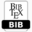## Using Fuzzy Artificial Neural Network TSK (Takagi, Sugeno, Kang) for Approximation and Prediction of Dissociation Energy of C-X-Bonds (X=F, Cl, Br, I) in Halogenated Hydrocarbons

 AUTHOR(S): Vladimir E. Tumanov, Elena S. Amosova, Bulat N. Gaifullin, Andrey I. Prokhorov TITLE Using Fuzzy Artificial Neural Network TSK (Takagi, Sugeno, Kang) for Approximation and Prediction of Dissociation Energy of C-X-Bonds (X=F, Cl, Br, I) in Halogenated HydrocarbonsPDFBIBTEX ABSTRACT The Takagi–Sugeno–Kang (TSK) fuzzy artificial neural network has been used for approximation of dissociation energies of C-X-bonds (X=F, Cl, Br, I) in halogenated hydrocarbons by the experimental data. Characteristics of molecule: electronegativity, force constant of the bond, the atom size of halogen served as variables. The comparison of predictions by the developed fuzzy network with the experimental data on the test sample is given. The obtained results are in good agreement with the experimental data. KEYWORDS fuzzy neural network, Takagi-Sugeno-Kang model, bond dissociation energy, halogenated hydrocarbon, electronegativity, bond force constant, halogen atom size REFERENCES  X1. Baskin I.I., Palyulin V.A., Zefirov N.S. Application of Artificial Neural Networks in Chemical and Biochemical Studies. Vestnik Moskovskogo Universiteta, Ser. 2. Khim. — 1999. Vol. 40(5). — P. 323-326 (Russ.).  X1. Baskin I.I., Palyulin V.A., Zefirov N.S. Application of Artificial Neural Networks in Chemical and Biochemical Studies. Vestnik Moskovskogo Universiteta, Ser. 2. Khim. — 1999. Vol. 40(5). — P. 323-326 (Russ.).  X2. Himmelblau D.M., Applications of Artificial Neural Networks in Chemical Engineering, Korean Z Chem. Eng., Vol. 17, N. 4, 2000, pp. 373-392.  X3. Gasteiger J., Zupan J., Neural networks in chemistry, Angev. Chem. Int. Ed. Engl., Vol. 32, 1993, pp. 503-527.  X4. Lazarev D. Yu., Tumanov V.E., Using of Artificial Neural Network to Assess the Bond Dissociation Energies of Organic Molecules, INORGANIC MATERIALS English translation of selected articles from Zavodskaya Laboratoriya. Diagnostika Materialov, Vol. 80, N. 5, 2014, pp. 74-76.  X5. Urata S., Takada A., Uchimaru T., Chandra A.K., Sekiya A., Artificial neural network study for the estimation of the C-H bond dissociation enthalpies, Journal of Fluorine Chemisty, Vol. 116, 2002, pp. 163-171.  X6. Tumanov V.E. Application of the Artificial Neural Networks and Fuzzy Logic for the Prediction of Reactivity of Molecules in Radical Reactions // In: Computational Problems in Engineering. Series: Lecture Notes in Electrical Engineering (Ed. N. Mastorakis, V. Mladenov.) — Switzerland: Springer International Publishing, Vol. 307, 2014, pp. 261-269.  X7. Lazarev D.Yu., Tumanov V.E., Prediction of bond dissociation energies of organic molecules by kinetic data of the radical reactions using the fuzzy knowledge base, BIT,s 3rd Annual Conference and EXPO of AnalytiX-2014, (April 25-28 2014. Dalian, China). 2014, p. 379.  X8. Tumanov V., Gaifullin G., Subjectoriented science intelligent system on physical chemistry of radical reactions, Modern Advances in Intelligent Systems and Tools, Vol. 431, 2012, pp. 121-126.  X9. Tumanov V.E., Bond dissociation energy of С-Х (X= F, Cl, Br, I) bonds in halogencontaining hydrocarbons: correlation ratios with electronegativity, force constants of bonds and radii of atom X, Butlerov Communications, Vol.40, N.8, 2015, pp. 140-146.  X10. Jang J., Sun C., Mizutani E., Neuro-fuzzy and Soft Computing, and, Prentice Hall 1997.  X11. Shinozaki N., Sibuya M., Tanabe K, Numerical algorithms for the Moore-Penrose inverse of a matrix: interative methods, Annals of the Institute of Statistical Mathematics, Vol. 24, N. 1,1972, pp. 621-629. Cite this paper Vladimir E. Tumanov, Elena S. Amosova, Bulat N. Gaifullin, Andrey I. Prokhorov. (2016) Using Fuzzy Artificial Neural Network TSK (Takagi, Sugeno, Kang) for Approximation and Prediction of Dissociation Energy of C-X-Bonds (X=F, Cl, Br, I) in Halogenated Hydrocarbons. International Journal of Mathematical and Computational Methods, 1, 146-148Copyright © 2016 Author(s) retain the copyright of this article.This article is published under the terms of the Creative Commons Attribution License 4.0